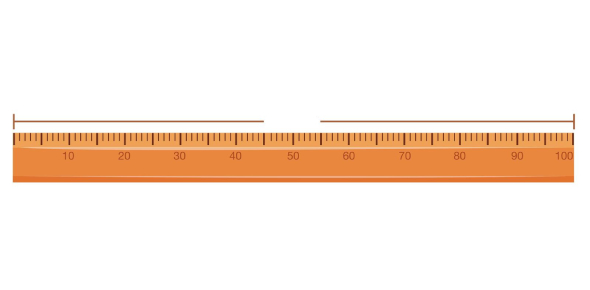# The Measurement Quiz

10 Questions | Attempts: 15665
ShareSettingsPlay the measurement quiz here to test your understanding of different measurements and how much you know about them. There are different scales for measurements, and there may be many that you may or may not be familiar with. After you take this quiz, your understanding of measurements will be even better. So, take this fun quiz and try to get a good score on this. Give your best in this!

• 1.
An inch is
• A.

Bigger than a centimeter

• B.

Smaller than a centimeter

• C.

Equal to a centimeter

• D.

None of these

• E.

None of these

• 2.
One inch is equal to
• A.

2cm

• B.

2.3cm

• C.

2.45cm

• D.

2.54cm

• 3.
Which of these is bigger than a millimeter?
• A.

Centimeter

• B.

Feet

• C.

Meter

• D.

All of these

• 4.
Temperature is measured in
• A.

Liter

• B.

Celsius

• C.

Ounce

• D.

None of these

• 5.
Liquid is measured in
• A.

Kilogram

• B.

Liter

• C.

Centimeter

• D.

Millimeter

• 6.
A ton is more than
• A.

A kilogram

• B.

An ounce

• C.

A gram

• D.

All of these

• 7.
1 feet is equal to ____ inches.
• A.

10

• B.

11

• C.

12

• D.

13

• 8.
1 kilogram is equal to
• A.

500 grams

• B.

750 grams

• C.

1000 grams

• D.

1200 grams

• 9.
A pound is _____ than a kilogram.
• A.

Less

• B.

More

• C.

Equal

• D.

None of these options is correct.

• 10.
Height can not be measured in
• A.

Meters

• B.

Liters

• C.

Centimeters

• D.

Feet

## Related TopicsBack to top
×

Wait!
Here's an interesting quiz for you.